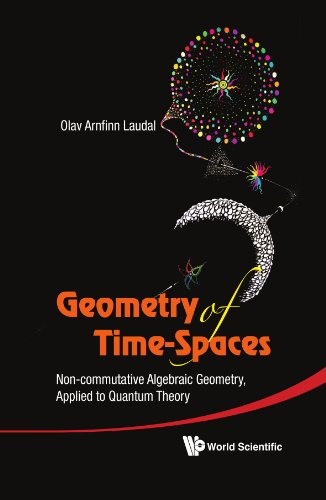# Geometry of Time-Spaces

Description

This is a monograph about non-commutative algebraic geometry, and its application to physics. The main mathematical inputs are the non-commutative deformation theory, moduli theory of representations of associative algebras, a new non-commutative theory of phase spaces, and its canonical Dirac derivation. The book starts with a new definition of time, relative to which the set of mathematical velocities form a compact set, implying special and general relativity. With this model in mind, a general Quantum Theory is developed and shown to fit with the classical theory. In particular the “toy”-model used as example, contains, as part of the structure, the classical gauge groups u(1), su(2) and su(3), and therefore also the theory of spin and quarks, etc.